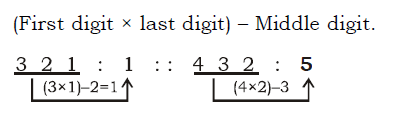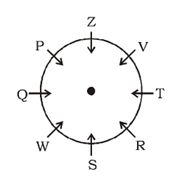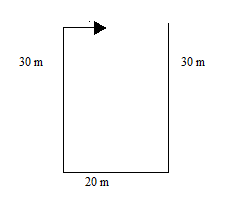# SSC CGL 2018 Practice Test Papers | Reasoning Ability (Day-37)

Dear Aspirants, Here we have given the Important SSC CGL Exam 2018 Practice Test Papers. Candidates those who are preparing for SSC CGL 2018 can practice these questions to get more confidence to Crack SSC CGL 2018 Examination.

[WpProQuiz 3250]

Click “Start Quiz” to attend these Questions and view Explanation

1) Select the related letters/ word/ number/ figure from the given alternatives.

321 : 1 : : 432 : ?

a) 5

b) 6

c) 9

d) 10

2) Select the related letters/ word/ number/ figure from the given alternatives.

APPLE: AEPPL::DUBAI: ?

a) AIUDB

b) UIDAB

c) BAIDU

d) IABDU

3) Find the odd word/ letters/ numbers/ figure from the given responses.

a) 6 : 16

b) 7 : 19

c) 8 : 27

d) 11 : 31

4) In a class of 60 students, A is 10th from top and B is 31st from bottom. 10 students are in between C and B. If some students, exactly in between C and A, go to park and others go to Zoo, then how many students went to Zoo?

a) 56

b) 54

c) 53

d) Cannot be determined

5) If R means ×, D means ÷, A means + and S means –, then the value of

95 D 19 R 11 S 28 A 17 is –

a) 30

b) 42

c) 44

d) 50

P, Q, R, S, T, V, W and Z are sitting around a circular table facing the centre but not necessarily in the same order. S is third to the right of P. P is second to the right of V. R is second to the left of V and third to the right of Q. W is not sitting adjacent to V. Z is second to the right of T.

6) Who is sitting to the next right to R?

a) S

b) T

c) Z

d) V

7) If W is related to V, S is related to Z then Q is related to which of the following?

a) P

b) R

c) T

d) S

8) What is the position of P with respect to V?

a) Immediate left

b) Second to the right

c) Third to the left

d) Fourth to the left

D walks 30 meters towards south then turns left and walks 20 meters. Again he turns right and walks 30 meters and then turns right and Walks for a certain distance and stops.

9) After stopping, in which direction is he facing?

a) West

b) East

c) North

d) South

10) Which of the following distance is cannot possible distance the between his starting point and ending point?

a) 35 m

b) 16 m

c) 10 m

d) 12 mVowels are arranged in as ascending order followed by constant letters

6 x 3 – 2 = 16

7 x 3 – 2 = 19

8 x 3 – 2 = 22

11 x 3 – 2 = 31

The position of C is unknown. So Answer cannot be determined.

95 ÷ 19 × 11 – 28 + 17

= 5 × 11 – 28 + 17

= 55 – 28 + 17

= 72 – 28

= 44

Direction (6-8)Direction (9-10)